# On-line Compression Model

On-line compression models are formally defined in Chapter 8 of Vovk et al. (2005). Such a model is a way of summarizing the information in the training set such that:

• the summary for any sequence of observations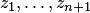can be computed by a known rule from its last element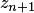and the summary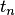of the preceding observations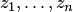;
• for any sequence of observations, the distribution of the pair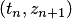, whereis the summary of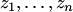, is known.

The method of conformal prediction works for any on-line compression model. Standard examples are the randomness assumption, the Gauss linear model, and the Markov model.

On-line compression models are an on-line version of Per Martin-Lof's and Steffen Lauritzen's repetitive structures; there is, essentially, a one-to-one correspondence between the two notions. Repetitive structures have served as a tool in standard statistical modelling. For the history of using repetitive structures in standard statistical modelling, see Vovk et al. (2005), Section 8.8. In many cases, the history predates the formal definition of repetitive structures by Martin-Lof in 1974: see, e.g., Gauss linear model and randomness assumption.

Relations between standard statistical modelling and on-line compression modelling (or repetitive structures) are the topic of an open problem.

The notion of an on-line compression model (and that of repetitive structure) can be argued to go back to Kolmogorov's programme of algorithmic foundations of probability: see Vovk et al. (2005), Section 8.8.

Bibliography

• Steffen Lauritzen (1988). Extremal Families and Systems of Sufficient Statistics. Springer, New York.
• Per Martin-Lof (1974). Repetitive structures. In Ole E. Barndorff-Nielsen, Preben Blaesild, and Geert Schou, editors, Proceedings of Conference on Foundational Questions in Statistical Inference, Aarhus. Memoirs 1.
• Vladimir Vovk, Alexander Gammerman, and Glenn Shafer (2005). Algorithmic learning in a random world. Springer, New York.